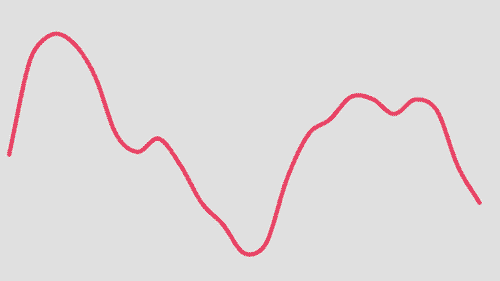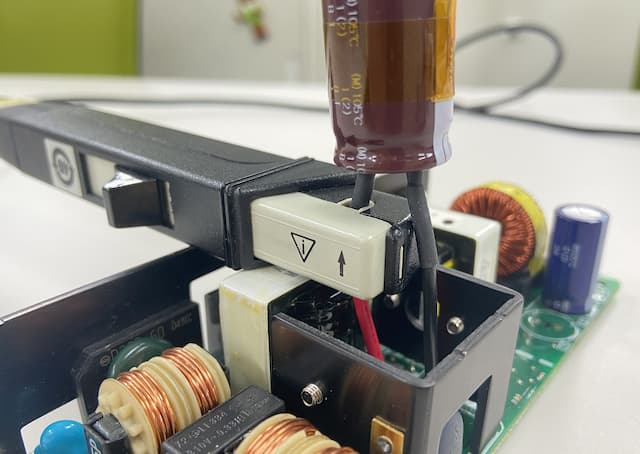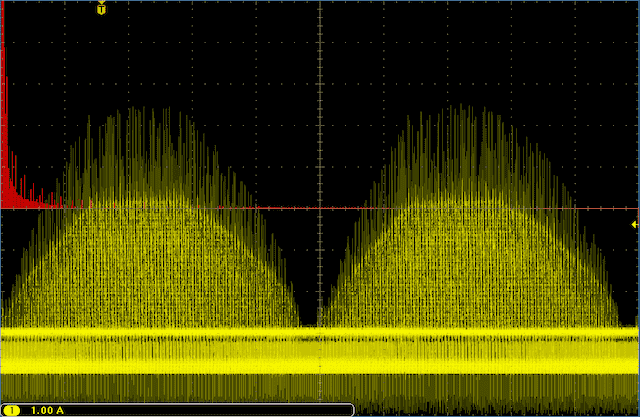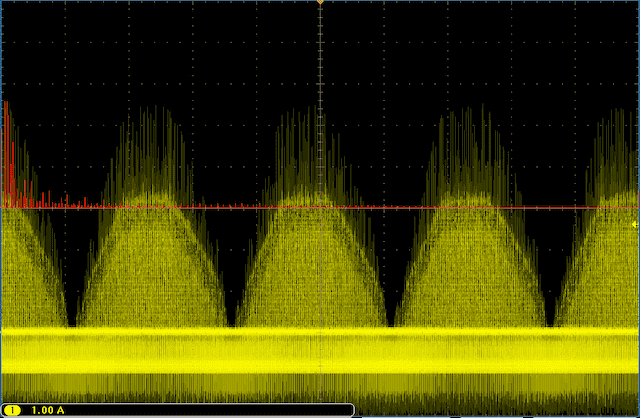### How To Measure Ripple Current Using the FFT Analysis Function

The FFT analysis function on an oscilloscope is useful when you want to measure the effective value of the ripple current, such as for use in calculating the endurance of an aluminum electrolytic capacitor.
The video below explains the actual process for using this function.
##### Example of Multiple Overlapping Frequency Components
When using an aluminum electrolytic capacitor in something such as a power factor correction (PFC) circuit, there are overlapping ripple currents ranging from low frequencies from the commercial power supply frequencies (100 Hz or 120 Hz) after rectification and high frequencies coming from the switching terminal.
DC/DC power supplies may also have overlapping ripple currents with multiple switching frequency components and complex frequency components coming from higher harmonics.
In these cases, it is quite difficult to calculate the effective value of the ripple current from the waveform.
##### FFT analysis
If your oscilloscope has an FFT analysis function, it is possible to calculate the effective value of the ripple current for each frequency. Then you can sum these values to find the effective value of the calculated ripple current for a specific frequency (specific frequency of rated ripple current, such as 120 Hz).
FFT stands for Fast Fourier Transform. When analyzing the frequency and effective value of the ripple current, this operation can be used to decompose the measured waveform into each frequency component and calculate the effective value for each.Concept of Fourier Transform

##### Procedure
【Key points for equipment selection】
• Oscilloscope
Use a model with a record length of 100k or more.
• Current Probes
Make sure to select the proper probe, either an AC probe or a DC probe, depending on the frequency range of the circuit.
• Extension cable
If you need to extend the capacitor leads when connecting the current probe, use a thick cable in order to reduce the effect on impedance.

【Oscilloscope operation】
1. Extend the capacitor terminals with cables and attach the current probe.2. Operate the circuit with the expected load conditions, and get an integral multiple of the waveform period to show on the oscilloscope.

Be aware that the ripple current following FFT transform may not be estimated correctly if it is not an integral multiple of the waveform period.Example of display showing an integral multiple of waveform periodExample of display not showing an integral multiple of waveform period
(The wave is cut off on the left and right)
3. Use the oscilloscope FFT operation function to switch from time domain display to frequency domain display.
4. The key point is to display in Linear RMS.

【Processing FFT analysis data】
1. Export data from the oscilloscope.
You may find it more convenient if you output the data in CSV format.
2. Open the data in spreadsheet software, and create a scatter plot from the frequencies on the x-axis and effective values of ripple current on the y-axis.
3. Read the peak value and frequency for the spectrum from the scatter plot.
This value will be the effective value of the ripple current at each frequency.
【Use in calculating capacitor heat generation】
Use the effective value of the ripple current calculated thus far to find the heat generated by the capacitor.
1. Use the frequency calibration coefficient to convert the effective value of the ripple current at each frequency measured during FFT analysis to the effective value of the ripple current at the rated frequency of the capacitor used (120 Hz or 100 kHz, depending on the series).
2. Generate the effective values of ripple current after frequency calibration.
3. Use the rated ripple current and measured values for frequency calibrated ripple current to calculate the value for heat generation.
The method for calculating the heat generation of a capacitor from the measured ripple current value is explained in-depth in our article on "Lifetime of Aluminum Electrolytic Capacitors".